# Abstract

The phenomenon of reflection and refraction is studied at the welded interface between two different porous solids. One is saturated with single non-viscous fluid and other is saturated with two immiscible viscous fluids. The incidence of Pf, Ps or SV wave through porous solid saturated with non-viscous fluid results in the three reflected waves and the four waves refracted to porous medium saturated with two immiscible viscous fluids. For the presence of viscosity in pore-fluids, the waves refracted to corresponding medium attenuate in the direction normal to the interface. It is also revealed that for the post-critical incidence of Ps wave, the reflected Pf and SV waves becomes evanescent and for the post-critical incidence of SV wave, the reflected Pf wave becomes evanescent. While, the occurrence of critical incidence is not observed for the incidence of Pf wave. The ratios of amplitudes of reflected and refracted waves to that of incident wave are expressed through a non-singular system of linear algebraic equations. These amplitude ratios are used further to calculate the shares of different scattered waves in the energy of incident wave. For a particular numerical model, the energy shares are computed for incident direction varying from normal incidence to grazing incidence. The conservation of energy across the interface is verified. Effects of non-wet saturation of pores, frequency of wave and porosity on the energy partitions are depicted graphically and discussed.

Keywords:
Reflection and refraction; porous solid; non-viscous; viscosity; critical angle; saturation; energy partition

# 1 INTRODUCTION

A mathematical model of the porous medium is intended to characterize the mechanical behaviour besides particle dynamics. A theory of porous media aims to explore the surprisingly large number of applications of porous materials. The most ideal area of application is geophysics. While petroleum geophysics or petrophysics, due to its economic importance, appears to monopolize the field of potential applications, one should not minimize the importance of other applications such as rock mechanics, soil mechanics and hydrogeology. The quantitative description of elastic wave propagation in a porous medium containing a single fluid is one of the classic problems in the physics of flow through porous materials. (Biot, 1956aBiot, M.A., 1956a. The theory of propagation of elastic waves in fluid-saturated porous solid, I. Low-frequency range. Journal of the Acoustical Society of America 28, 168-178., bBiot, M.A., 1956b. The theory of propagation of elastic waves in fluid-saturated porous solid, II. High-frequency range. Journal of the Acoustical Society of America 28, 179-191.; 1962aBiot, M.A., 1962a. Mechanics of deformation and acoustic propagation in porous media. Journal of Applied Physics 33, 1482-1498., bBiot, M.A., 1962b. Generalized theory of acoustic propagation in porous dissipative media; Journal of Acoustical Society of America 34, 1254-1264.) derived the fundamental equations for the study of wave motion in porous solids saturated with a compressible fluid. One additional but slow dilatational wave has been the main feature of wave propagation in such materials. Confirmation of Biot's theory came a bit late. (Plona, 1980Plona, T.J., 1980. Observation of a second bulk compressional wave in a porous medium at ultrasonic frequencies. Applied Physics Letters 36(4), 259-261.) and (Berryman, 1981Berryman, J. G., 1981. Elastic wave propagation in fluid saturated porous media. Journal of the Acoustical Society of America 69, 416-424.) conducted experiments to observe this slow dilatational wave.

An extension of the theory to include the effects of two immiscible pore fluids on the behavior of elastic waves was proposed long ago by Brutsaert (Brutsaert, 1964Brutsaert, W., 1964. The propagation of elastic waves in unconsolidated unsaturated granular mediums. Journal of Geophysical Research 69, 243-257., Brutsaert and Luthin, 1964Brutsaert, W., Luthin, J.N., 1964. The velocity of sound in soils near the surface as a function of the moisture content. Journal of Geophysical Research 69(4), 643-652.) as a generalization of the seminal (Biot, 1962Biot, M.A., 1962a. Mechanics of deformation and acoustic propagation in porous media. Journal of Applied Physics 33, 1482-1498.) poroelasticity model for a single-fluid system. Literature is extensive on mixture theories. Other notable references are (Morland, 1972Morland L.W., 1972. A theory of slow fluid through a porous media. Journal of Geophysical Research 77, 890-900.), (Bedford and Drumheller, 1978Bedford, A., Drumheller, D.S., 1978. A variational theory of immiscible mixtures. Arch. Rational Mech. Anal. 68, 37-51), (Bowen, 1980Bowen, R.M., 1980. Incompressible porous media models by use of theory of mixtures. International Journal of Engineering Science 18, 1129-1148., 1982Bowen, R. M., 1982. Compressible porous media models by use of theory of mixtures. International Journal of Engineering Science 208, 697-735.). (Hassanizadeh and Gray, 1990Hassanizadeh, S.M., Gray W.G., 1990. Mechanics and thermodynamics of multiphase flow in porous media including interphase boundaries. Advances in Water Resources 13(4), 169-186.) have shown that Biot's theory and mixture theory are equivalent, if Biot's parameter for fluid-solid coupling is neglected. Mixture theory for porous media saturated by fluids includes the concept of volume fraction to characterize the microstructure of the medium. (Bedford and Drumheller, 1983Bedford, A., Drumheller, D.S., 1983. Theories of immiscible and structured mixtures. International Journal of Engineering Science 21, 863-960.) gave an extensive survey of continuum theories of mixtures of immiscible constituents. In later years, (Santos et al., 1990aSantos, J.E., Carbero, J., Douglas, J. Jr., 1990a. Static and dynamic behaviour of a porous solid saturated by a two phase fluid. Journal of the Acoustical Society of America 87(4), 1428-1438., bSantos, J.E., Douglas, J. Jr., Cobero, J., Louvera, O.M., 1990b. A model for wave propagation in a porous medium saturated by a two phase fluid. Journal of the Acoustical Society of America 87, 1439-1448.) derived the governing equations and presented a method to calculate elastic constants for isotropic porous solids saturated by two fluids. Then the credit for comprehensive discussion on wave propagation in porous solids saturated with multiphase fluids goes to (Garg and Nayfeh, 1986Garg, S.K. and Nayfeh, A.H., 1986. Compressional wave propagation in liquid and/or gas saturated elastic porous media. Journal of Applied Physics 60, 3045-3055.), (Corapcioglu and Tuncay, 1996Corapcioglu, M.Y., and K. Tuncay. 1996. Propagation of waves in porous media. Advances in Porous Media, Elsevier Science Publishers, Amsterdam, The Netherlands, 361-441.), (Tuncay and Corapcioglu, 1997Tuncay, K., Corapcioglu, M.Y., 1997. Wave propagation in poroelastic media saturated by two fluids. Journal of Applied Mechanics 64, 313-319.) and (Lo et al., 2005Lo, W.-C., Sposito, G., Mayer, E., 2005. Wave propagation through elastic porous media containing two immiscible fluids. Water Resources Research 41(2), W02025, (20 pp.).).

Phenomenon of reflection and refraction of waves from the boundaries of poroelastic materials has been discussed by many researchers, for example, (Deresiewicz, 1960Deresiewicz, H., 1960, The effect of boundaries on wave propagation in a liquid-filled porous solid-I: Reflection of plane waves at a free plane boundary (Non Dissipative Case), Bulletin of the Seismological Society of America 50, 599-607., 1964aDeresiewicz, H., 1964a, The effect of boundaries on wave propagation in a liquid-filled porous solid. VI. Love waves in a double surface layer. Bulletin of the Seismological Society of America 54, 417-423., bDeresiewicz, H., 1964b, The effect of boundaries on wave propagation in a liquid-filled porous solid. VII. Surface waves in a half-space in the presence of liquid layer. Bulletin of the Seismological Society of America 54, 425-430.), (Deresiewicz and Rice, 1962Deresiewicz, H., and Rice, J.T., 1962, The effect of boundaries on wave propagation in a liquid-filled porous solid-III: Reflection of plane waves at a free plane boundary (General Case), Bulletin of the Seismological Society of America 52, 595-625.), (Dutta and Ode, 1983Dutta, N.C., and Ode, H., 1983, Seismic reflection from a gas-water contact, Geophysics, 48, 148-162.), (Sharma and Gogna, 1991Sharma, M.D., Gogna, M.L., 1991. Seismic wave propagation in a viscoelastic porous solid saturated by viscous liquid. Pure and Applied Geophysics 135(3), 383-400.), (Sharma and Saini, 1992Sharma, M.D., Saini, T., 1992, Pore alignment between two dissimilar saturated poroelastic media: Reflection and refraction at the interface, International Journal of Solids and Structures 29(11), 1361-1377.), (Sharma, 2008Sharma, M.D., 2008. Wave propagation across the boundary between two dissimilar poroelastic solids, Journal of Sound and Vibration 314(3), 657-671.), (Lin et al., 2005Lin, C. H., Lee, V.W., Trifunac, M. D., 2005. The reflection of plane waves in a poroelastic half-space saturated with inviscid fluid. Soil Dynamics and Earthquake Engineering 25, 205-223.), (Tomar and Arora, 2006Tomar, S.K., Arora, A., 2006. Reflection and transmission of elastic waves at an elastic/porous solid saturated by two immiscible fluids. International Journal of Solids and Structures 43, 1991-2013.), (Arora and Tomar, 2007Arora, A., Tomar, S.K., 2007. Elastic waves at porous/porous elastic half-spaces saturated by two immiscible fluids. Journal of Porous Media 10 (8), 751-768.), (Yeh et al., 2010Yeh, C.-L., Lo, W.-C., Jan, C.-D., Yang, C.-C., 2010. Reflection and refraction of obliquely incident elastic waves upon the interface between two porous elastic half-spaces saturated by different fluid mixtures. Journal of Hydrology 395, 91-102.), (Markov, 2009Markov. M.G., 2009, Reflection of Elastic Waves at Fluid-Fluid Interfaces in Porous Media. Izvestiya, Physics of the Solid Earth, 2009, Vol. 45, No. 9, pp. 769-776.), (Sharma and Kumar, 2011Sharma, M.D., Kumar, M., 2011. Reflection of attenuated waves at the surface of a porous solid saturated with two immiscible viscous fluids, Geophysical Journal International 184(1), 371-384.), (Kumar and Saini, 2012Kumar, M., Saini, R., 2012. Reflection and refraction of attenuated waves at the boundary of elastic solid and porous solid saturated with two immiscible viscous fluids. Applied Mathematics Mechanics 33(6), 797-816.), (Sharma and Saini, 2012Sharma, M.D., Saini, R., 2012. Wave propagation in porous solid containing liquid filled bound pores and two-phase fluid in connected pores. Europeon Journal of Mechanics A/Solids 36, 53-65.), (Kumar and Sharma, 2013Kumar M., Sharma M.D., 2013. Reflection and transmission of attenuated waves at the boundary between two dissimilar poroelastic solids saturated with two immiscible viscous fluids. Geophysical Prospecting 61(5), 1035-1055.), (Kumar and Kumari, 2014Kumar M., Kumari. M., 2014. Reflection of attenuated waves at the surface of a fractured porous solid saturated with two immiscible viscous fluids. Latin American Journal of Solids and Structures 11(7), 1206-1237.). Present problem considers to study the reflection and refraction phenomenon at a plane interface between a non-viscous porous solid saturated with single fluid and a porous solid saturated by two immiscible fluids. The fluid-saturated porous solid is modeled with the classical Biot's theory and fluids-saturated porous solid is described by the poroelasticity theory of (Tuncay and Corapcioglu, 1997Tuncay, K., Corapcioglu, M.Y., 1997. Wave propagation in poroelastic media saturated by two fluids. Journal of Applied Mechanics 64, 313-319.). The field equations of classical Biot's theory and poroelasticity theory of (Tuncay and Corapcioglu, 1997Tuncay, K., Corapcioglu, M.Y., 1997. Wave propagation in poroelastic media saturated by two fluids. Journal of Applied Mechanics 64, 313-319.) are solved for harmonic propagation of three longitudinal waves and one transverse wave in porous medium considered. The work presented here considers the reflection and refraction phenomena at poroelastic/poroelastic interface without any restriction on incidence angle. Porous medium saturated with two immiscible viscous fluids is considered dissipative due to the presence of viscosity in pore-fluids. Hence, the waves refracted to dissipative porous medium are identified as inhomogeneous waves with attenuation always normal to the interface.

# 2 FUNDAMENTAL THEORY

## 2.1 Review of Biot's Theory

Let u' and U'f be the displacement vectors of the matrix and the interstitial fluid respectively. According to Biot's theory (1956Deresiewicz, H., 1960, The effect of boundaries on wave propagation in a liquid-filled porous solid-I: Reflection of plane waves at a free plane boundary (Non Dissipative Case), Bulletin of the Seismological Society of America 50, 599-607., 1962aDeresiewicz, H., 1964a, The effect of boundaries on wave propagation in a liquid-filled porous solid. VI. Love waves in a double surface layer. Bulletin of the Seismological Society of America 54, 417-423., bDeresiewicz, H., 1964b, The effect of boundaries on wave propagation in a liquid-filled porous solid. VII. Surface waves in a half-space in the presence of liquid layer. Bulletin of the Seismological Society of America 54, 425-430.), the equations of motion for fluid-saturated homogeneous poroelastic solid, in the absence of dissipation, are

(1)(2)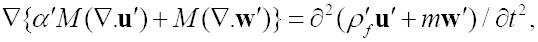where w' refer to the relative displacement vector of the fluid with respect to the solid matrix measured in volume per unit area, i.e., w' = β'(U'f - u'), λ', μ' = Lame's constant for the solid, ρ' and ρ'f are the mass densities of the bulk material and fluid respectively, m is Biot's parameter which depends upon porosity β' density ρ'f , α' and M are elastic constants related to the coefficients of jacketed and unjacketed compressibilities.

The constitutive equations for the isotropic porous solid are written as follows

(3)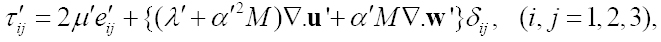(4)where τ'ij are components of the total stress on a representative element of volume of the solid skeleton, e'ij are the components of strain in skeleton and τ'f is the pressure in pore fluid.

Considering the Helmholtz resolution of each of the two displacement vectors in the form

(5)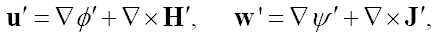where Φ', H' are the potential functions of the solid phase of porous medium, and ψ', J' are the potential functions of flow of the pore fluid relative to the solid. Inserting these expressions of u' and w' into (equations 1)-(2), we obtain the following equations

(6)(7)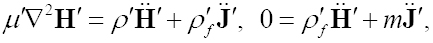where P = λ' + 2μ' + α'2M, Q = α'M and dots over these scalars and vectors denote partial time derivatives.

For two-dimensional propagation of harmonic waves in the x-z plane, we assume

(8)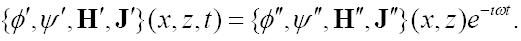Substituting ϕ' and ψ' into (equation 6), and eliminating ψ" then P -wave equations for solid frame become

(9)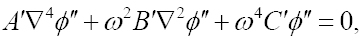where A' = PM - Q2, B' = mP + ρ'M - 2ρ'f Q,C' =ρ'2f.

(Equation. 9) can be decomposed into

(10)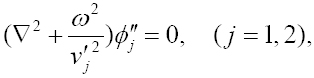where, (j = 1,2).

Here, (Equation 10) shows that two P-waves exist in the medium. The wave corresponding to ϕ1" is called fast P (or Pf) wave propagating with phase velocity v1' and the wave corresponding to ϕ2" is called slow P (or Ps) wave propagating with phase velocity v2'. Therefore, the general solution of P-waves for the solid and fluid phase are given by

(11)(12)where j = 1, 2)., (and

Substituting H' and J' into (equation 7), and eliminating J", then S-wave equations for solid frame is obtained as

(13)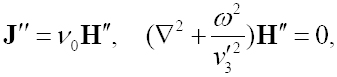where equation 13) defines the existence of a transverse wave propagating with phase velocity v3'.The (and

The general displacements of the solid and fluid in the x-z lane are given by

(14)(15)where H' = (H')y and J' = (J')y = v0H'.

## 2.2 Governing Equations for Fluids-Saturated Porous Solid

Following (Tuncay and Corapcioglu, 1997Tuncay, K., Corapcioglu, M.Y., 1997. Wave propagation in poroelastic media saturated by two fluids. Journal of Applied Mechanics 64, 313-319.), the equations of motion in the absence of body forces for low frequency wave propagation in a porous elastic medium saturated by gas and liquid, are defined as follows:

(16)(17)(18)The coefficients aij denote elastic constants and are given by

a11 = Kfr, a12 = a21 = Ksαsσ(al + Kl)/D, a13 = a31 = Klαs (1 - σ)(al + Kg)/D,

a22 = Ksαs (σKl + al)/D, a23 = a32 = KgKlσαl/D, a33 = Klαl (Kg (1 - σ) + al)/D,

D = Kg (1 - σ) + al + Klσ, dg = vgα2g /(Ξ0Ξrg), dl = vlα2l /(Ξ0Ξrl),

where us , ug and ul are the displacement vectors in porous elastic solid, gas and liquid phases respectively. Dots over these vectors denote partial time derivatives. Kg and Kl are the bulk moduli of gas and liquid phases respectively whereas Kfr is bulk modulus of the porous frame or drained matrix. Gfr is the shear modulus of porous solid. αs , αg and αl are the volume fractions of the solid, gas and liquid phases respectively. ‹ρs›, ‹ρg›, and ‹ρl› are the volume-averaged densities of porous solid, gas and liquid phases respectively. Si = αi/Φ, (i = g, l) and Si = αi/Φ, (i = g, l) with σ + Si = 1 and al = Kcapσ(1 - σ), Kcap is equivalent to bulk modulus for macroscopic capillary pressure (Garg and Nayfeh, 1986Garg, S.K. and Nayfeh, A.H., 1986. Compressional wave propagation in liquid and/or gas saturated elastic porous media. Journal of Applied Physics 60, 3045-3055.). Φ porosity of the porous media. dg and dl are the dissipation coefficients of gas and liquid phases, respectively. These coefficients involve relative permeabilities (Ξrg, Ξrl) and viscosities (vg, vl) of the corresponding phases and the intrinsic permeability of the composite medium (Ξ0).

Following (Tuncay and Corapcioglu, 1997Tuncay, K., Corapcioglu, M.Y., 1997. Wave propagation in poroelastic media saturated by two fluids. Journal of Applied Mechanics 64, 313-319.), then stress in porous solid is given by

(19)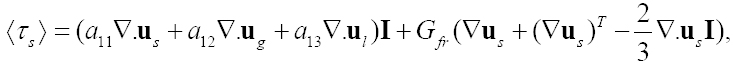and pressures in fluids are given by

(20)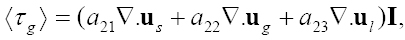(21)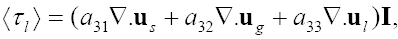where I is unit tensor.

Through the usual Helmholtz resolution of a vector, the displacement vectors can be conveniently written in the following form

(22)(23)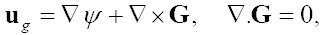(24)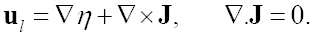Inserting these values of us, ug and ul into (equations 16)-(18), we obtain the following equations

(25)(26)(27)(28)(29)(30)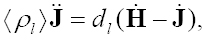where a*11 = a11 + 4/3Gfr and dots over these scalars and vectors denote partial time derivatives.

For two-dimensional propagation of harmonic waves in x-z plane, we assume

(31)where ω denotes angular frequency of the vibration of constituent particles in porous aggregate.

Substituting these values of ϕ, ψ and η in (25), (26) and (27), yields

(32)(33)(34)where χg = ldg, χl = ldl , Λs = ‹ρs› + χg + χl, Λg = ‹ρ g› + χg, Λl = ‹ρl› + χl.

The (equations 33) and (34) of this system are solved into two relations, given by

(35)(36)Using these relations into (equation 32), we obtain

(37)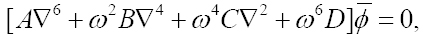where

A = a*11A3 + a12A1 + a13A2, B = a*11B3 + ΛsA3 + a12B 1 + a13B2 - χgA1 - χ1A2,

C = a*11C3 + ΛsB3 + a12C1 + a13C2 - χgB1 - χlB2, D = ΛsC3 - χgC1 - χlC2,

A1 = a23a13 - a12a33, A2 = a12a23 - a13a22, A3 = a22a33 - a23a23,

B1 = a33χg - a12Λl - a23χl, B2 = a22χl - a13Λg - a23χg, B3 = a22Λl + a33Λg,

C1 = Λl χg, C2 = Λg χl, C3 = Λg Λl.

The solution of (equation 37) is written as

(38)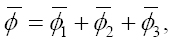Where

(39)The solutions of (equation 39) correspond to the three longitudinal waves. The waves corresponding to i = 1, 2, 3) being the three longitudinal waves propagating with phase velocities vi (i = 1, 2, 3) respectively. Using the (equation 38) in (31), we get(

(40)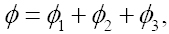(41)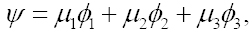(42)whereSimilarly, we assume

(43)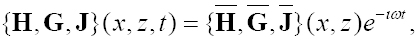and subsisting relations (43) into (equations 28)-(30), we get

(44)(45)where.

By substituting relations (45) into (equation 44), we get

(46)where equation 46) defines the existence of a transverse wave propagating with phase velocity v4. For two-dimensional motion in the x-z plane, displacement of solid and fluid phases are given by. The (

(47)(48)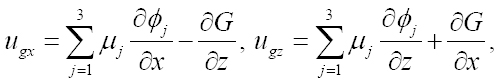(49)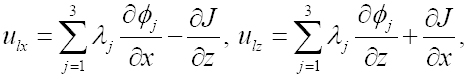where H = (H)y, G = (G)y = TgH and J = (J)y = TlH.

# 3 FORMULATION OF THE PROBLEM

We consider a non-viscous porous solid half-space saturated with single fluid (impervious) and a porous solid half-space saturated by two immiscible viscous fluids chosen as gas and liquid in welded contact along a plane interface. Rectangular Cartesian coordinate system (x, y, z) is chosen with the plane of interface as z = 0 and the Z-axis is pointing into the porous elastic solid half-space so that, the non-viscous porous solid half-space saturated with only fluid (medium I) occupies the region -∞ < z < 0 and porous half-space saturated with two immiscible fluids (medium II) occupies the region 0 < z < ∞. Our aim is to study a reflection and refraction problem in two dimensional x-z plane and the incident wave is assumed to be incident obliquely at the interface, after through the non-viscous porous solid half-space saturated with single fluid.

# 4 BOUNDARY CONDITIONS

We assume that two half space separated by a plane interface along z = 0 are in perfect contact. Therefore, the boundary conditions are the continuity of stress components and displacement components along the interface plus one more condition which restrict the flow of two fluids of porous solid into non-viscous porous solid saturated with single fluid, i.e., at z = 0.

(50)where superposed dots denote the partial time derivatives.

# 5 REFLECTION AND REFRACTION OF WAVES AT A PLANE INTERFACE

We consider only two-dimensional reflection and refraction problem, in the (x, z)-plane with incident waves assumed to originate in the non-viscous porous solid saturated with single fluid (medium I). The incident wave is assumed to originate in medium I and become incident at the plane interface z = 0, making an angle θ0 with the z. It results in three reflected waves (Pf, Ps and SV) in medium I and refracted as four inhomogeneous plane waves (P1, P2, P3 and SV) in the fluids saturated porous solid. For medium I, a set of such plane wave solutions for the displacement potentials for reflected waves, satisfying (10) and (13) are given by

(51)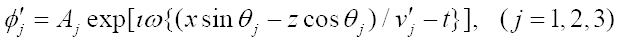where ϕ'3 is replacing H' (to maintain the uniformity of the symbols) in the relations (14) and (15) and the arbitrary constant (A1, A2, A3) denotes the amplitudes of reflected Pf ,Ps and SV waves, respectively.

Displacement potentials for the incident wave are as follows

1. (i) for incident Pf wave

(52)1. (ii) for incident Ps wave

(53)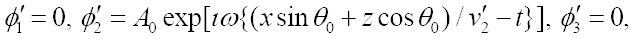1. (iii) for incident SV

(54)Following (Borcherdt, 1982Borcherdt, R. D., 1982. Reflection-refraction of general P-and type-I S-waves in elastic and anelastic solids. Geophysical Journal of the Royal Astronomical Society 70(3), 621-38.), the plane wave solutions for the displacement potentials satisfying (39) and (46) are

(55)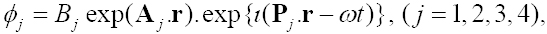where ϕ4 is replacing H (to maintain the uniformity of the symbols) in the relations (47)-(49). The coefficients Bj , (j = 1, 2, 3, 4), denotes the amplitudes of the refracted P 1, P2, P3 and SV waves, respectively. The propagation vectors (Pj) and attenuation vectors (Aj) are defined by

(56)with definitions

(57)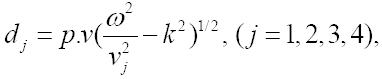where p.v. in (57) denotes the principal value in the square root of complex quantity enclosed. k is a complex number with kR > 0 to ensure propagation in positive x-direction. The subscripts R and I denote the real and imaginary parts of the corresponding complex quantities. In terms of the angle yj between propagation vector and attenuation vector and angle of refraction (θ'j) in medium II, complex wave number k can be written as

(58)Making the use of potentials, given by (51) and (55), the boundary conditions are satisfied through the Snell's law, given by

(59)and

(60)which implies that γj = θ'j, (j = 1, 2, 3, 4) i.e., waves in porous solid with twin fluid attenuating in z-direction. v0 = v'j is used for incident wave identified with 'j' in porous medium with single fluid.

In addition to (equations 59) and (60), the amplitude ratios Zj of reflected Pf, reflected Ps, reflected SV, refracted P1, refracted P2, refracted P3 and refracted SV waves to that of incident wave should satisfy the system of seven non-homogeneous equations represented as

(61)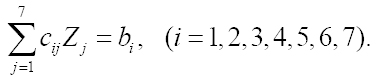The coefficients cij are as follows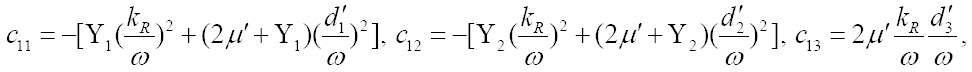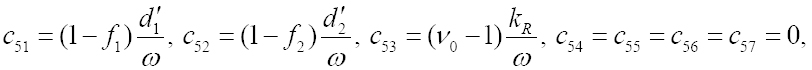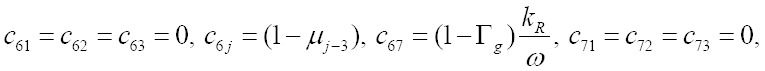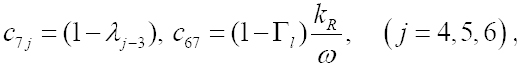whereThe constant terms b1 on the right hand side of (equations 61) are given by

1. (i) for incident Pf wave

b1 = -c11, b2 = c21, b3 = -c31, b4 = c41, b5 = c51, b6 = 0, b7 =0,

1. (ii) for incident Ps wave

b1 = -c12, b2 = c22, b3 = -c32, b4 = c42, b5 = c52, b6 = 0, b7 =0,

1. (iii) for incident SV wave

b1 = c13, b2 = -c23, b3 = c33, b4 = -c43, b5 = c53, b6 = 0, b7 = 0.

We now consider a surface element of unit area at the interface between two media. Purpose is to calculate the partition of energy (of the incident wave) among the reflected and refracted waves on the both sides of this surface. Following (Achenbach, 1973Achenbach, J.D., 1973. Wave Propagation in Elastic Solids. North-Holland, Amsterdam.), the rate at which the energy is transferred per unit area of the surface is given by the scalar product of surface traction and the particle velocity, denoted by P*. The time average of P* over a period, denoted by ‹P*›, represents the average energy transmission per unit surface area per unit time. Thus, on the surface with normal along z-direction, the average energy intensities of the waves in the fluid saturated porous solid are defined by

(62)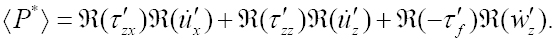We have ‹ℜ(f) · ℜ(g)› = 1/2 ℜ(f· ḡ), for two arbitrary complex functions f and g. This relation is used to calculate the energy ratios giving the rate of average energy transmission of all the reflected and refracted waves to that of incident wave.

Expressions for these energy ratios Ei (i = 1, 2, 3) for the reflected Pf, Ps, SV waves, respectively, are given by

(63)where ‹Pi *› = {λ' + 2μ' + M'(α' + fi )}|Zi |2i = 1,2),for (and

1. (i) for incident Pf wave1. (ii) for incident Ps wave1. (iii) for incident SV wave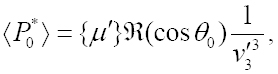which are the energy intensities of the incident Pf, Ps, SV waves, respectively.

For the porous solid saturated with twin fluid, the average energy intensities of the waves on the surface with normal along z-direction, are defined by

(64)and evaluated as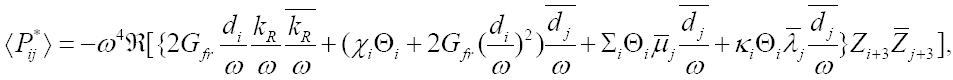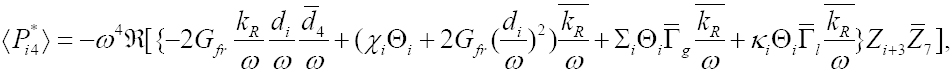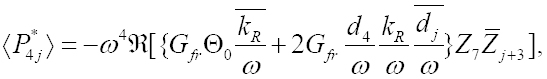whereand,

Σi = a12 + μja22 + λja23, κi = a13 + μja23 + λja33, (i = 1, 2, 3).

An energy matrix

(65)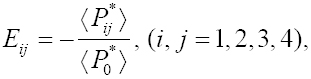calculates the distribution of energy among the four waves traveling into the dissipative porous medium saturated by two immiscible fluids. Solving the system of (equations 61), by Gauss elimination method, provides the complex unknowns Zi (i = 1, 2, ..., 7), which are used further in relations (63) and (65) to calculate the energy ratios Ei (i = 1, 2, 3) and Eij (i, j = 1, 2, 3, 4), respectively.

The diagonal entries of energy matrix Eij represent the energy share of the four refracted waves in the medium. Terms E11, E 22, E33, E44 are identified as the refraction (energy) coefficients for P1, P1, P3, SV waves, respectively. Sum of all non-diagonal entries of this energy matrix gives the share of interaction energy among all the refracted waves in the medium. This part of energy, given by ERR = E1 + E2 + E3 + E11 + E22 + E33 + E44 + ERR = 1, yields the conservation of incident energy across the interface, through the relation

# 6 NUMERICAL RESULTS AND DISCUSSION

## 6.1 Numerical Example

The derivations for velocities, amplitude ratios and energy ratios involve a large number of parameters. Then, in order to study the dependence of amplitude and energy ratios on the direction of oblique incidence, we confine our numerical work to a particular model. Keeping in view the availability of numerical data, we consider the model consisting of a reservoir rock (sandstone) saturated with water and CO2 is chosen for the numerical model of porous medium (Garg and Nayfeh, 1986Garg, S.K. and Nayfeh, A.H., 1986. Compressional wave propagation in liquid and/or gas saturated elastic porous media. Journal of Applied Physics 60, 3045-3055.) in welded contact with water-saturated sandstone which is assumed to be an impervious porous solid.

The elastic and dynamical constants for the dry porous are given by, Kfr = 12Gpa, Gfr = 9Gpa, αs = 0.8, ‹ρs › = 2120kg/m3. A part (αg) of the pore volume is occupied by CO2 gas of bulk modulus Kg = 3.7MPa and partial density ‹ρg › = σ(1 - αs )103kg/m3. With bulk modulus Kl = 2.7GPa and partial density ‹ρl › = (1 - αs )(1 - σ)990kg/m3, water is the other pore-fluid that occupies the remaining pore volume. The value of Kcap = 0.1MPa is used to represent capillary pressure. The values chosen for dissipation coefficient are dg = 0.04MPa - sec/m2 and d1 = 1MPa - sec/m2.

The elastic and dynamical constants for the water-saturated sandstone are given by

λ' = 3.034Gpa, μ' = 9.22Gpa, M = 8.87Gpa, ρ' = 2170kg/m3, ρ'f = 1000kg/m3,

m = 3731kg/m3, α' = 0.3227, β' = 0.268.

## 6.2 Reflection and Refraction Coefficients

The energy of incident wave is shared among the three reflected (Pf, Ps, SV) waves, four refracted (P 1, P2, P3, SV) waves interaction energy. Due to the inhomogeneous propagation of refracted waves, a part of the refracted energy share identified as the interaction energy. The variations in energy partition with incident direction are presented in Fig. 1-3 (for incident Pf wave), Fig. 4-6 (for incident Ps wave), Fig. 7-9 (for incident SV wave) and are discussed as follows.

For incident Pf wave:

The variations of energy shares of three reflected (Pf, Ps, SV) waves, four refracted (P1, P2, P3, SV) waves and interaction energy with θ0 ∈ (0,90º) are exhibited in Fig. 1, for three different values of σ = 0.01, 0.5, 0.99. Values chosen for other parameters are Kcap = 0.05Kl , ω/2π = 0.1kHz, Φ = 0.45. Near normal incidence, for any σ, only reflected Ps and refracted P1 waves have larger energy shares. On the other hand, at grazing incidence for any σ, only the reflected Pf wave has a significant energy share. A considerable variation in energy shares is visible with the change of gas share in pores. It is noted that with the change of gas share in pores the variation pattern of the energy share of reflected SV wave is analogous to the refracted SV wave. However, the response of reflected Pf wave to this change is nearly opposite to the reflected Ps wave. A comparison among the energy shares of reflected and refracted waves implies that the existence propagation of dilatational (i.e.,P2, P3) waves is just namesake. Interaction energy may not have a physical significance but it ensures conservation of incident energy and certifies, by default, the correctness of whole analytic part of the procedure. The negative (positive) sign of interaction energy implies the travel of energy towards (away from) the interface.

Figure 1
Energy shares of reflected (Pf, Ps, SV) waves, refracted (P1, P2, P3, SV) and interaction among refracted waves; variations with incident direction (θ0) and gas share in pores (σ); Kcap = 0.05Kl, ω/2π = 0.1kHz, Φ = 0.45; incident Pf wave.

Fig. 2 exhibits the variations of energy shares of three reflected (Pf, Ps, SV) waves, four refracted (Pf, Ps, SV) waves and interaction with θ0 ∈ (0.90º), for three different frequencies ω/2π = 0.1,1,5kHz. Values chosen for other parameters are Kcap = 0.05Kl , σ = 0.5kHz, Φ = 0.45. Kcap = 0.05Kl, σ = 0.5kHz, Φ = 0.45. The effect of wave frequency ω is clearly visible on all the energy shares. It is also noted that the effect of frequency is nearly same to the effect of gas share in pores except the incidence direction at which the change starts in energy partitions with the change in wave frequency.

Figure 2
Energy shares of reflected (Pf, Ps, SV) waves, refracted (P1, P2, P3, SV) and interaction among refracted waves; variations with incident direction (θ0) and frequency of wave ω; Kcap = 0.05Kl, σ = 0.5, Φ = 0.45; incident Pf wave.

Fig. 3 exhibits the variations of energy shares of three reflected (Pf, Ps, SV) waves, four refracted (P1, P2, P3, SV) waves and interaction energy with θ0 ∈ (0.900), for three different values of Φ = 0.25, 0.35, 0.45. Values chosen for other parameters are Kcap = 0.05Kl, σ = 0.5kHz, ω/2π = 1kHz. Similar to the effect of σ in Fig. 1 and ω in Fig. 2, the significant effect of Φ on energy partition is observed. In this case, a larger porosity may be responsible for stronger refracted (P2, P3) waves.

Figure 3
Energy shares of reflected (Pf, Ps, SV) waves, refracted (P1, P2, P3, SV) and interaction among refracted waves; variations with incident direction (θ0) and porosity (Φ) Kcap = 0.05Kl, ω/2π = 1kHz, σ = 0.5; incident Pf wave.

For incident Ps wave:

The variations of energy shares of three reflected (Pf, Ps, SV) waves, four refracted (P1, P2, P3, SV) waves and interaction energy with θ0 ∈ (0.900) are exhibited in Fig. 4, for three different values of σ = 0.01, 0.5, 0.99. Near normal incidence, for any σ, only reflected Pf and refracted P1 waves have larger energy shares. On the other hand, at grazing incidence for any σ, only the reflected Ps wave has a significant energy share.

Figure 4
The same as the Fig. 1 but for incident Ps wave.

A significant variation in energy shares is visible with the change of gas share in pores. It is quite evident that in the incidence of Pf wave, the critical angles for the reflected Pf and SV waves are observed around θ0 = 280 and θ0 = 440, respectively, for any value of σ. A comparison among the energy shares of reflected and refracted waves shows, a relatively insignificant refracted P2 wave strengthens a lot for σ = 0.01. It is inferred from plot that reflected SV wave is very little sensitive to the change in σ. However, the response of the refracted SV wave to this change is significant.

Fig. 5 exhibits the variations of energy shares of three reflected (Pf, Ps, SV) waves, four refracted (P1, P2, P3, SV) waves and interaction energy with θ 0 ∈ (900), for three different frequencies ω/2π = 0.1,1.5kHz. The effect of wave frequency (ω) is clearly visible on all the energy shares. It is also noted that the effect of frequency is nearly same to the effect of gas share in pores except the incidence direction at which the change starts in energy partitions with the change in wave frequency.

Figure 5
The same as the Fig. 2 but for incident Ps wave.

Fig. 6 exhibits the variations of energy shares of three reflected (Pf, Ps, SV) waves, four refracted (P1, P2, P3, SV) waves and interaction energy with θ 0 ∈ (900), for three different values of Φ = 0.25, 0.35, 0.45 Similar to the effect of σ in Fig. 4 and ω in Fig. 5, the significant effect of Φ on energy partition is observed. In this case, a larger porosity may be responsible for stronger refracted (P2, P3) waves. A comparison of the energy shares of various reflected and refracted waves implies that the contribution of refracted (P2, P3) waves to the total wave-field is just a namesake, for any value of Φ.

Figure 6
The same as the Fig. 3 but for incident Ps wave.

For incident SV wave:

The variations of energy shares of three reflected (Pf, Ps, SV) waves, four refracted (P1, P2, P3, SV) waves and interaction energy with θ0 ∈ (900) are exhibited in Fig. 7, for three different values of σ = 0.01, 0.5, 0.99 Near normal incidence, for any σ, only refracted SV wave have larger energy shares. On the other hand, at grazing incidence for any σ, only the reflected SV wave has a significant energy share. A significant variation in energy shares is visible with the change of gas share in pores. It is quite evident that in the incidence of Pf wave, the critical angle for the reflected Pf wave is observed round θ0 = 420, for any value of σ. The response of reflected SVwave to this change with incident direction is nearly opposite to the refracted SV wave.

Figure 7
The same as the Fig. 1 but for incident SV wave.

It is clearly visible from the plot that energy share of reflected Pf wave became weakens with the increase of gas share in pores for the incidence up to an angle around θ0 = 350 While, energy share of refracted P1 wave strengthens with the increase of gas share in pores for the incidence up to an angle around θ0 = 350. Near the critical incidence, a peak in energy shares of reflected Pf and refracted P1 waves are observed with the change of gas share in pores.

Fig. 8 exhibits the variations of energy shares of three reflected (Pf, Ps, SV) waves, four refracted (P1, P2, P3, SV) waves and interaction energy for θ 0 ∈ (900), with three different frequencies ω/2π = 0.1,1.5kHz. The effect of wave frequency (ω) is clearly visible on all the energy shares. It is also noted that the effect of frequency is nearly same to the effect of gas share in pores except the incidence direction at which the change starts in energy partitions with the change in wave frequency.

Figure 8
The same as the Fig. 2 but for incident SV wave.

Fig. 9 exhibits the variations of energy shares of three reflected (Pf, Ps, SV) waves, four refracted (P1, P2, P3, SV) waves and interaction energy for θ 0 ∈ (900), with three different values of Φ = 0.25, 0.35, 0.45. Similar to the effect of σ in Fig. 7 and ω in Fig. 8, the significant effect of Φ on energy partition is observed. In this case, a larger porosity may be responsible for stronger refracted (P2, P3) waves. A comparison of the energy shares of various reflected and transmitted waves implies that the contribution of refracted (P2, P3) waves to the total wave-field is just a namesake, for any value of Φ.

Figure 9
The same as the Fig. 3 but for incident SV wave.

# 7 CONCLUSIONS

The presented work is the theoretical analysis of the phenomena of reflection and refraction at the welded interface between a non-viscous porous solid saturated with single fluid and a porous solid saturated with two immiscible viscous fluids. There are three reflected and four refracted waves for given incident wave. Partition of incident energy among the reflected waves, refracted waves are calculated along with the interaction energy. The interaction comes from the interaction among refracted waves. Reflection-refraction phenomenon is studied for incidence of three waves, that is Pf, Ps and SV. The variations in reflection/refraction energy coefficients are analyzed for a particular numerical model with variations in gas share in pores, frequency of incident wave and porosity of medium. Some interesting observations from the numerical example may be important and hence are explained as follows.

• Conservation of the incident energy is obtained for the presence of interaction energy due to the interference between refracted waves. This certifies the correctness of all the analytic derivations which form the complete procedure.

• At the normal incidence of Pf wave, the energy is shared mainly among reflected Ps and refracted P1 waves. While at the grazing incidence of Pf wave, domination shifts in favor of the reflected Pf wave.

• At the normal incidence of Ps wave, the energy is shared mainly among reflected Pf and refracted P1 waves. While at the grazing incidence of Ps wave, domination shifts in favor of the reflected Ps and SV waves. While at the grazing incidence of SV wave, the reflected SV wave dominates over all other scattered waves.

• For the incidence of Ps wave, the energy share of refracted P2 wave is of diagnostic importance for a minute presence of gas in pores.

• For the incidence of Ps wave, the critical angles are observed for reflected Pf and SV waves. On the other hand for the incidence of SV wave, critical angle is observed only for reflected Pf wave.

• The effect of gas share in pores, wave frequency and porosity on energy partitions are observed for all the reflected and refracted waves.

# Acknowledgments

One of the authors (RS) acknowledges the financial support of CSIR, New Delhi (India), in form of SRF through the grant number 09/105 (0169)/2008-EMR-1.

# References

• Achenbach, J.D., 1973. Wave Propagation in Elastic Solids. North-Holland, Amsterdam.
• Arora, A., Tomar, S.K., 2007. Elastic waves at porous/porous elastic half-spaces saturated by two immiscible fluids. Journal of Porous Media 10 (8), 751-768.
• Bedford, A., Drumheller, D.S., 1978. A variational theory of immiscible mixtures. Arch. Rational Mech. Anal. 68, 37-51
• Bedford, A., Drumheller, D.S., 1983. Theories of immiscible and structured mixtures. International Journal of Engineering Science 21, 863-960.
• Berryman, J. G., 1981. Elastic wave propagation in fluid saturated porous media. Journal of the Acoustical Society of America 69, 416-424.
• Biot, M.A., 1956a. The theory of propagation of elastic waves in fluid-saturated porous solid, I. Low-frequency range. Journal of the Acoustical Society of America 28, 168-178.
• Biot, M.A., 1956b. The theory of propagation of elastic waves in fluid-saturated porous solid, II. High-frequency range. Journal of the Acoustical Society of America 28, 179-191.
• Biot, M.A., 1962a. Mechanics of deformation and acoustic propagation in porous media. Journal of Applied Physics 33, 1482-1498.
• Biot, M.A., 1962b. Generalized theory of acoustic propagation in porous dissipative media; Journal of Acoustical Society of America 34, 1254-1264.
• Borcherdt, R. D., 1982. Reflection-refraction of general P-and type-I S-waves in elastic and anelastic solids. Geophysical Journal of the Royal Astronomical Society 70(3), 621-38.
• Bowen, R. M., 1982. Compressible porous media models by use of theory of mixtures. International Journal of Engineering Science 208, 697-735.
• Bowen, R.M., 1980. Incompressible porous media models by use of theory of mixtures. International Journal of Engineering Science 18, 1129-1148.
• Brutsaert, W., 1964. The propagation of elastic waves in unconsolidated unsaturated granular mediums. Journal of Geophysical Research 69, 243-257.
• Brutsaert, W., Luthin, J.N., 1964. The velocity of sound in soils near the surface as a function of the moisture content. Journal of Geophysical Research 69(4), 643-652.
• Corapcioglu, M.Y., and K. Tuncay. 1996. Propagation of waves in porous media. Advances in Porous Media, Elsevier Science Publishers, Amsterdam, The Netherlands, 361-441.
• Deresiewicz, H., 1960, The effect of boundaries on wave propagation in a liquid-filled porous solid-I: Reflection of plane waves at a free plane boundary (Non Dissipative Case), Bulletin of the Seismological Society of America 50, 599-607.
• Deresiewicz, H., 1964a, The effect of boundaries on wave propagation in a liquid-filled porous solid. VI. Love waves in a double surface layer. Bulletin of the Seismological Society of America 54, 417-423.
• Deresiewicz, H., 1964b, The effect of boundaries on wave propagation in a liquid-filled porous solid. VII. Surface waves in a half-space in the presence of liquid layer. Bulletin of the Seismological Society of America 54, 425-430.
• Deresiewicz, H., and Rice, J.T., 1962, The effect of boundaries on wave propagation in a liquid-filled porous solid-III: Reflection of plane waves at a free plane boundary (General Case), Bulletin of the Seismological Society of America 52, 595-625.
• Dutta, N.C., and Ode, H., 1983, Seismic reflection from a gas-water contact, Geophysics, 48, 148-162.
• Garg, S.K. and Nayfeh, A.H., 1986. Compressional wave propagation in liquid and/or gas saturated elastic porous media. Journal of Applied Physics 60, 3045-3055.
• Hassanizadeh, S.M., Gray W.G., 1990. Mechanics and thermodynamics of multiphase flow in porous media including interphase boundaries. Advances in Water Resources 13(4), 169-186.
• Kumar M., Kumari. M., 2014. Reflection of attenuated waves at the surface of a fractured porous solid saturated with two immiscible viscous fluids. Latin American Journal of Solids and Structures 11(7), 1206-1237.
• Kumar M., Sharma M.D., 2013. Reflection and transmission of attenuated waves at the boundary between two dissimilar poroelastic solids saturated with two immiscible viscous fluids. Geophysical Prospecting 61(5), 1035-1055.
• Kumar, M., Saini, R., 2012. Reflection and refraction of attenuated waves at the boundary of elastic solid and porous solid saturated with two immiscible viscous fluids. Applied Mathematics Mechanics 33(6), 797-816.
• Lin, C. H., Lee, V.W., Trifunac, M. D., 2005. The reflection of plane waves in a poroelastic half-space saturated with inviscid fluid. Soil Dynamics and Earthquake Engineering 25, 205-223.
• Lo, W.-C., Sposito, G., Mayer, E., 2005. Wave propagation through elastic porous media containing two immiscible fluids. Water Resources Research 41(2), W02025, (20 pp.).
• Markov. M.G., 2009, Reflection of Elastic Waves at Fluid-Fluid Interfaces in Porous Media. Izvestiya, Physics of the Solid Earth, 2009, Vol. 45, No. 9, pp. 769-776.
• Morland L.W., 1972. A theory of slow fluid through a porous media. Journal of Geophysical Research 77, 890-900.
• Plona, T.J., 1980. Observation of a second bulk compressional wave in a porous medium at ultrasonic frequencies. Applied Physics Letters 36(4), 259-261.
• Santos, J.E., Carbero, J., Douglas, J. Jr., 1990a. Static and dynamic behaviour of a porous solid saturated by a two phase fluid. Journal of the Acoustical Society of America 87(4), 1428-1438.
• Santos, J.E., Douglas, J. Jr., Cobero, J., Louvera, O.M., 1990b. A model for wave propagation in a porous medium saturated by a two phase fluid. Journal of the Acoustical Society of America 87, 1439-1448.
• Sharma, M.D., 2008. Wave propagation across the boundary between two dissimilar poroelastic solids, Journal of Sound and Vibration 314(3), 657-671.
• Sharma, M.D., Gogna, M.L., 1991. Seismic wave propagation in a viscoelastic porous solid saturated by viscous liquid. Pure and Applied Geophysics 135(3), 383-400.
• Sharma, M.D., Kumar, M., 2011. Reflection of attenuated waves at the surface of a porous solid saturated with two immiscible viscous fluids, Geophysical Journal International 184(1), 371-384.
• Sharma, M.D., Saini, R., 2012. Wave propagation in porous solid containing liquid filled bound pores and two-phase fluid in connected pores. Europeon Journal of Mechanics A/Solids 36, 53-65.
• Sharma, M.D., Saini, T., 1992, Pore alignment between two dissimilar saturated poroelastic media: Reflection and refraction at the interface, International Journal of Solids and Structures 29(11), 1361-1377.
• Tomar, S.K., Arora, A., 2006. Reflection and transmission of elastic waves at an elastic/porous solid saturated by two immiscible fluids. International Journal of Solids and Structures 43, 1991-2013.
• Tuncay, K., Corapcioglu, M.Y., 1997. Wave propagation in poroelastic media saturated by two fluids. Journal of Applied Mechanics 64, 313-319.
• Yeh, C.-L., Lo, W.-C., Jan, C.-D., Yang, C.-C., 2010. Reflection and refraction of obliquely incident elastic waves upon the interface between two porous elastic half-spaces saturated by different fluid mixtures. Journal of Hydrology 395, 91-102.

# Publication Dates

• Publication in this collection
July 2016